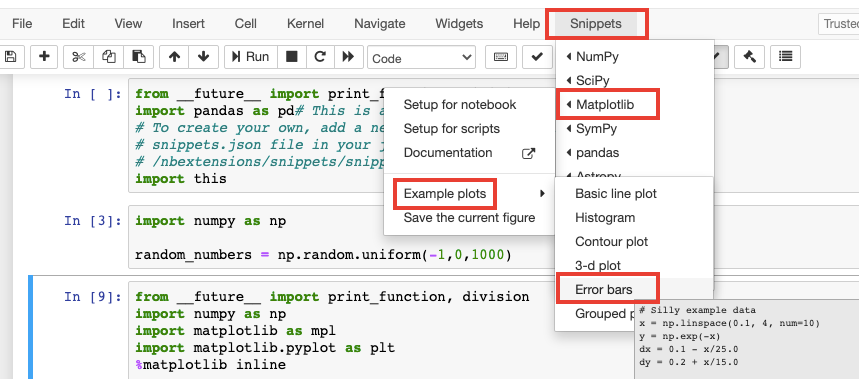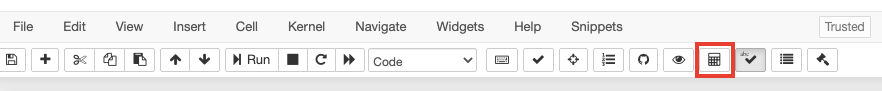top of page
Search
•Magdalena Konkiewicz

# Jupyter Notebook Extensions (part 2)Image by Bessi from Pixabay

Introduction

This article is a follow-up to my previous article that has introduced you to Jupyter notebook extensions and has presented my favorite ones. If you have not read it yet you probably should start there as it introduces the general concepts such as:

1) what are Jupyter notebook extensions,

2) how to install them,

3) and presents some basic but very useful extensions.

The list of extensions demonstrated in my previous article is not exhaustive so the goal of the current article is to add a few other, more complex but still very beneficial extensions to the previous list.

Let's get started.

This a very useful extension that allows you to run the code against the current kernel without the need to modify the original notebook cells. The extension allows you to launch a scratchpad cell where you can run all additional checkups.

Have you ever end up doing some additional code checkups while running the notebook like checking a value of the list at a particular index or printing the size of the output and then needing to delete this code? Scratchpad is ideal for that.

Once you have enabled the extension you can find a scratchpad toggle button in the bottom right-hand corner or use the 'ctrl + b' keyboard shortcut to launch it.Once triggered you should see the scratchpad on the right-hand side with the yellow background. In the screenshot below I have my main notebook cell where I produce 1000 random numbers between -1 and 0, and I use scratchpad to check the first 10 values.This is an ideal extension for beginners and for people who are a bit lazy and would rather avoid a lot of typing. The extension allows you to insert ready code snippets into your cells with some basic functionalities for popular scientific packages such as pandas, NumPy, matplotlib, or scipy. It also has some basic python and markdown code examples.

Once you have enabled Snippets Menu you should see the Snippets appearing in your Jupyter Notebook menu just after the Help button.If you open the Snippets menu you will see that there are quite a lot of snippets to choose from and they are organized by package names.

Let's try some of them. We will set up the notebook to use matplotlib first. Just go to Snippets -> Matplotlib -> Setup for notebook.This should insert the following code in the cell.

```from __future__ import print_function, division
import numpy as np
import matplotlib as mpl
import matplotlib.pyplot as plt
%matplotlib inline```

After that let's see an example of the error bar code provided. In order to achieve this navigate to Snippets -> Matplotlib -> Example plots -> Error bars.This code snippet should insert the example code for plotting error bars with matplotlib package.

```# Silly example data
x = np.linspace(0.1, 4, num=10)
y = np.exp(-x)
dx = 0.1 - x/25.0
dy = 0.2 + x/15.0

# Make the plot
plt.figure()
plt.errorbar(x, y, xerr=dx, yerr=dy)
plt.title(r"Title here (remove for papers)")
plt.xlabel(r"Description of \$x\$ coordinate (units)")
plt.ylabel(r"Description of \$y\$ coordinate (units)")
plt.show()```

Once you run the cell you should see the following graph.You have just created the plot with error bars in less than 1 minute. Now you can customize it with your own data.

Initialization Cells

Another very useful extension that allows you to set up the cells that initialize the whole notebook. This would be usually the cells with imports and ones that load data files and do some basic data frame formatting so you have access to well-formatted data.

Once you installed the extension you need to enable it within the menu by going to View -> Cell Toolbar -> Initialization Cell.Once you have done it you will see that each cell will have a tick box named 'Initialization Cell' in the top right-hand corner. Tick them if you want the cell to become an initialization one.Once you start the notebook all initialization cells are run automatically. You can also toggle the run of initialization cells from the jupyter notebook menu using the icon shown in the screenshot below.Split cells notebook

This an extension that helps with the organization of your notebook. It allows you to split the cells so some of them could be displayed next to each other instead of being positioned one below another. This is useful when you want to compare some code in parallel, for example inspecting two data frames.

Once you have installed the extension you can use the 'shift + s' keyboard shortcut when in command mode to split the cell. Command mode can be entered using the escape button on the active cell.

For example, if you had the code inspecting two different data frames similar to two code snippets below. Each snippet creates a data frame with random numbers and calls a describe() function.

```import pandas as pd
df_1 = pd.DataFrame(np.random.randint(0,100,size=(100, 4)), columns=list('ABCD'))
df_1.describe()```

```df_2 = pd.DataFrame(np.random.randint(0,100,size=(100, 4)), columns=list('ABCD'))
df_2. describe()```

You could use split cell functionality to achieve this kind of view.This would allow you to compare results side by side!

Summary

This was a list of some additional extensions that I use when working with Jupyter notebooks. I hope you will take an advantage of some of their functionality in your next projects.

Happy Coding!